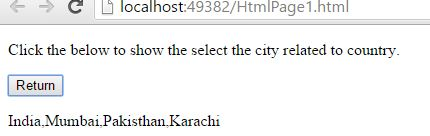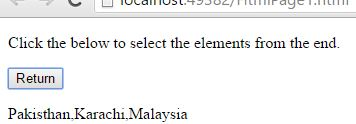# Slice Array in JavaScript

#### Array Slice

The Array slice {`slice()` method} in JavaScript returns the  selected elements in an array, as a new array object. The array slice extracts the section of an array and returns the new array. The array slice has two parameters `slice(Start, End)`. The parameters 'Start' and 'End' values should be the numbers, either it may be positive numbers or negative numbers. Positive numbers selects the elements from the start of an array, where as negative numbers select the elements from the end of an array. The selection of elements in an array includes the 'Start' value, but does not includes the 'End' value.

NOTE: The Start parameter value must and should smaller than the End parameter value. The parameter values must be either positive or negative.

In JavaScript, by using Array slice {`slice()` method} we can return the selected elements in an array.

EXAMPLE: Returning the selected elements in an array by giving positive values to 'Start' and 'End' parameters.

```<p>Click the below to show the select the city related to country.</p>
<input type="button" onclick="myValue()" value="Return"/>
<p id="myId"></p>

<script>
function myValue() {
var a = ["India", "Mumbai", "Pakisthan", "Karachi", "Malaysia", "Abudhabi"];
var b = a.slice(0, 4);
document.getElementById("myId").innerHTML = b;
}
</script>
```

In the above code snippet we have given `Id` as "`myId`"to the second `<p>` element in the HTML code. There is a function myValue() in the`<script>`block which is connected to the onclick of the HTML button and array with value "["India", "Mumbai", "Pakisthan", "Karachi", "Malaysia", "Abudhabi"]" to the variable `a`. We need to return the selected elements in an array, for that we are using `slice()` method, which is commonly called as 'Array Slice', and we are giving the values '0' and '4' to `slice(0, 4)` method, that means it returns from the element '0' (Zero is the starting number when we are counting the elements in an array) to element '3'. Onclick of the button "Return" in the HTML code fires the function myValue() in the `<script>` block at the same time `slice()` method selects elements in an array as per the given input values and returns in an new array.

OUTPUTIn the above output we can notice that, the output includes the Start value '0',  but does not includes the End value '4'

(India=0, Mumbai=1, Pakistan=2, Karachi=3, Malaysia=4, Abudhabi=5)

NOTE: While counting the elements in an array, the count starts from zero (0). The positive input values to the `slice()` method parameters selects the elements from the start of an array.

EXAMPLE: Returning the selected elements in an array by giving negative values to 'Start' and 'End' parameters.

```<p>Click the below to select the elements from the end.</p>
<input type="button" onclick="myValue()" value="Return"/>
<p id="myId"></p>

<script>
function myValue() {
var a = ["India", "Mumbai", "Pakisthan", "Karachi", "Malaysia", "Abudhabi"];
var b = a.slice(-4, -1);
document.getElementById("myId").innerHTML = b;
}
</script>
```

Select elements using negative input values

In the above code snippet we have given negative input values to `slice()` method parameters. Onclick of the button "Return" in the HTML code fires the function myValue() in the `<script>` block at the same time `slice()` method selects elements in an array as per the given input values and returns in an new array.

OUTPUTNOTE: Negative input values to the `slice()` method parameters selects the elements from the end of an array.

Views: 3384 | Post Order: 158